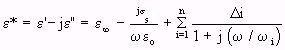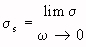### 4.3. TABULATED SUMMARY OF MEASURED VALUESThis section contains a summary of the measured values of dielectric properties of biological substances as a function of frequency and temperature, as reported in primary sources in the literature. Further information can be found in the listed references.
Tables 4.7 and 4.8 give the dielectric constant and conductivity values of different animal tissues, at indicated temperatures and frequencies. The temperature coefficient of the dielectric constant and conductivity of various body tissues are shown in Tables 4.9 and 4.10

#### Table 4.10. Temperature Coefficient Of Conductivity Of Various Body Tissues(Schwan, 1954)

The complex permittivity is generally frequency dependent and at a frequency, can be described by the Debye equation, similar to Equation 4.1 (Schwan and Foster, 1980; Foster et al., 1980):- (Equation 4.24)

where= relative-permittivity change due to dispersion associated withFrom Equation 4.24, we get- (Equation 4.25)

and

-(Equation 4.26)

Hurt (1985) has written a Fortran program that performs a least-squares fit of Equations 4.25 and 4.26 to permittivity data. Figures 4.12 and 4.13 are the curves for relative dielectric permittivity and conductivity, respectively, that result from fitting a 5-term Debye relation to muscle data (Tables 4.7 and 4.8). For muscle,is set equal to 4.3 (Grant et al., 1978; Hill, 1963).

The complex dielectric data in Tables 4.7-4.10 are from in vitro measurements of permittivity on excised human or animal tissues. Interest in reexamining tissue-permittivity values based on in vivo measurements has been increasing (Toler and Seals, 1977). With the in vivo procedure, a short monopole antenna is inserted into living tissue, and changes in the terminal impedance of the antenna are measured (Burdette et al., 1980). These impedance values can then be related to the complex permittivity of tissue.

#### Figure 4.13. Conductivity for muscle (W. D. Hurt, private communication, USAF School of Aerospace Medicine, 1985).

Figures 4.14-4.16 compare in vivo data for dielectric constants and conductivity with in vitro data; Figures 4.17-4.19 give additional in vivo data. These figures show only slight differences between the in vivo and in vitro values, except in the case of canine fat tissue (Figure 4.17) where the in vivo dielectric constant values are a factor of approximately 1.5-2 times the reported in vitro results above 100 MHz. These differences in the dielectric constant are attributed primarily to possible differences in water content between the in vivo and in vitro measurement conditions (Burdette et al., 1980). Conductivity values are also generally higher than in vitro values found in the literature (Schwan, 1957).

#### Figure 4.19. Measured values of relative dielectric constant and conductivity of rat blood at 23°C (Burdette et al., 1980).

Figures 4.20-4.24 show recently obtained in vivo data for several feline tissues at frequencies between 10 MHz and 1 GHz (Stuchly et al., 1981). These figures show significant differences in the properties of different types of the same tissue (e.g., skeletal and smooth muscle).

#### Figure 4.24. Relative permittivity of cat brain: tissue 1 -- gray matter; tissue 2 -- gray matter, 3 mm thick over white matter; tissue 3 -- white matter (Stuchly et al., 1981).

At frequencies below 100 kHz, a strong conductance anisotropy exists in muscle tissue (Rush et al., 1963). Data for anisotropic permittivity at low frequencies can be found in the works of Rush et al. (1963), Schwan (1957), and Johnson et al. (1975). Figure 4.25 shows the real part of the dielectric constant and conductivity of muscle tissue as a function of frequency for the parallel and perpendicular orientations (Epstein and Foster, 1983).

#### Figure 4.25. The real part of the dielectric constant (a) and the conductivity (b) of the canine skeletal muscle tissue at 37°C as a function of frequency, in parallel orientation (open and closed circles) and perpendicular orientation (crosses and triangles), averaged over five measurements on different samples, The dotted lines are the data for non-oriented muscle tissue (Epstein and Foster , 1983).

Figures 4.26-4.28 show the real and imaginary parts of the dielectric constant and the conductivity of ocular tissue at 37°C as a function of frequency (Gabriel et al., 1983). Figures 4.29 and 4.30 compare the dielectric constant and conductivity of the normal and tumor mouse tissue as a function of frequency (Rogers et al., 1983).

#### Figure 4.30. Conductivity of normal and tumor mouse tissue as a function of frequency (Rogers et al., 1983).mouse muscle,KHT fibrosarcoma tumor,RIF/I fibrosarcoma tumor.Go to Chapter 5.1